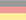# Constructive numbers

What I would like to present here as constructive numbers is based on a calculus by Jeffrey M. James, which he had presented in his master thesis "A Calculus of Number Based on Spatial Forms" from 1993. This in turn builds on ideas of John Horton Conway, George Spencer-Brown, Louis H. Kauffman and William Bricken auf. Using only three different brackets, James' calculus generates a very powerful number base with many interesting properties, starting from three simple axioms. It is not practical to do an accounting with the minimalistic notation without any syntactic sugar, but calculation rules and laws are made visible in a vivid and almost meditative way.

The calculus uses three different brackets. A round one for the e-function, a square one for the natural logarithm ln(x) and a angle one for the inversion. The nested tree structures constructed in this way use the drawing background as an empty space, so to speak, which is given a structure by their boundary drawings. Since e0 is equal to 1, if one allows only round brackets for the e-function, and does not allow to nest the brackets, one obtains a calculus for the natural numbers. If one additionally allows angle brackets for the inversion, which may be nested with the round brackets, one obtains the integers. Together with square brackets for the natural logarithm, which may only be nested alternately with the round brackets, whereby there are still no restrictions for the angle brackets, the rational numbers result. One could also describe these as arbitrarily nested multiplications and inversions of integers. If we additionally allow exponentiations with arbitrarily nested bases and rational exponents, we get the algebraic numbers.

If, with the exception of ln(0) with again two restrictions, one releases all nesting possibilities, one obtains an infinite distributive lattice of transcendental field extension, each with countably infinite degree of transcendence.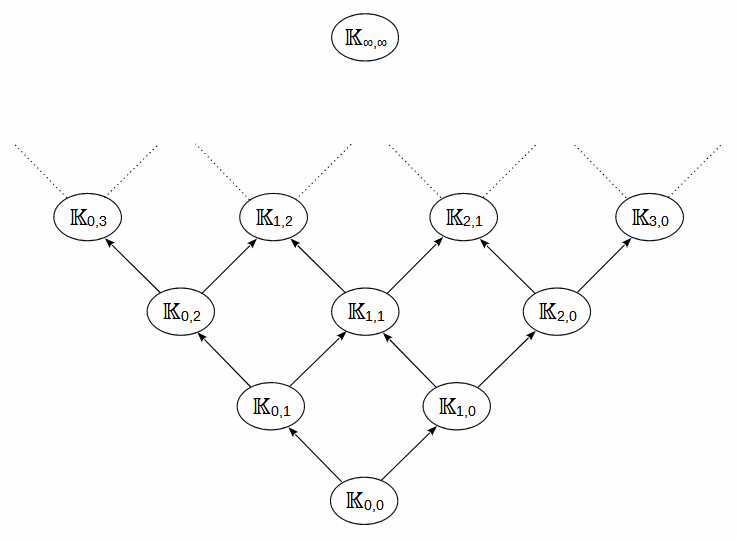𝕂0,0 = 𝔸
𝕂0,1 = 𝔸({ln k | k ∈ 𝔸\0})
𝕂1,0 = 𝔸({ek | k ∈ 𝔸})
𝕂0,2 = 𝕂0,1({ln k | k ∈ 𝕂0,1\0})
𝕂1,1 = 𝕂0,1({ek | k ∈ 𝕂0,1}) = 𝕂1,0({ln k | k ∈ 𝕂1,0\0})
𝕂2,0 = 𝕂1,0({ek | k ∈ 𝕂1,0})
...
𝕂n,m+1 = 𝕂n,m({ln k | k ∈ 𝕂n,m\0})
𝕂n+1,m = 𝕂n,m({ek | k ∈ 𝕂n,m})
...
𝕂∞,∞

The smallest field of the lattice 𝕂0,0 is equal to the algebraic numbers and the largest is 𝕂∞,∞. In it, e-functions and natural logarithms can be nested potentially infinitely many times. e would be element in all fields containing at least 𝕂1,0 and ee in all from 𝕂2,0. π would be element in all fields containing at least 𝕂0,1, ln(π) in all from 𝕂0,2 and eπ in all from 𝕂1,1. However, there is still no proof that e and π are algebraically independent, even though it looks very much like it. But even if they were, in the extreme case only the transcendence degrees within the lattice would be smaller. All fields of the lattice have countably infinite power, since a simple algorithm could iteratively construct all elements. Because of this, my suggestion would be to name the number fields as constructive numbers. Real numbers are overcountably infinite compared to these. Thus there are real numbers which lie outside of 𝕂∞,∞. These could be called destructive numbers. However, it is already difficult to prove the irrationality or transcendence of certain numbers. Probably, proving the destructiveness of numbers is even more difficult. The first number for which the proof of its transcendence succeeded in 1844 is a Liouville number. To my mind, it is a hot candidate for a number that is not only transcendent but even destructive.

```f1(x) = x
f2(x) = -x
f3(x) = ex
f4(x) = ln x
f3(f4(x)) = x   eln x = x
f4(f3(x)) = x   ln ex = x

<>     - Inversion        -0 = 0
() = o - Instanziation    e0 = 1
[] = ∎ - Abstraction    ln 0 = -∞ + [-∞...∞]i  (complete ambiguity in the imaginary part)

[(a)] = ([a]) = a     Involution                                 Axiom 1     ln ea = eln a = a
(a[bc]) = (a[b])(a[c])  Distribution of a                          Axiom 2     ea + ln (b + c) = ea + ln b + ea + ln c
a<a> =               Inversion of a                             Axiom 3     a - a = 0
<<a>> = a             Inverse Cancellation  (double Inversion)   Theorem 1   -(-a) = a
<a><b> = <ab>          Inverse Collection                         Theorem 2   (-a) + (-b) = -(a + b)

Proof of Inverse Cancellation     for a != ∎
<<a>>
<<a>><a>a   -Inversion of a
a   Inversion of <a>

Proof of Inverse Collection     for a,b != ∎
<a><b>
<a><b>ab<ab>   -Inversion of ab
<ab>   Inversion of a and b

Proof of additive self-inversion of void (ambiguous sign)   -0 = 0
<>
Inversion of void

Cardinality of a (aggregation second degree)
a = ([a]) = ([a][o])             -Involution2, -Involution1
aa = ([a][o])([a][o]) = ([a][oo]) 2x a, -Distribution of [a]
a...a = ([a][o...o])                 induction step over n ∈ ℕ

a * b    =>  ([a][b])
a / b    =>  ([a]<[b]>)     for b !=

Proof of Associativity of multiplication   (a*b)*c = a*(b*c)
([([a]  [b])][c]  )
(  [a]  [b]  [c]  )   Involution1
(  [a][([b]  [c])])   -Involution1

Proof of multiplicative self-inversion of o   1/1 = 1
(<[o]>)
(<   >)   Involution1
(     )   Inversion of void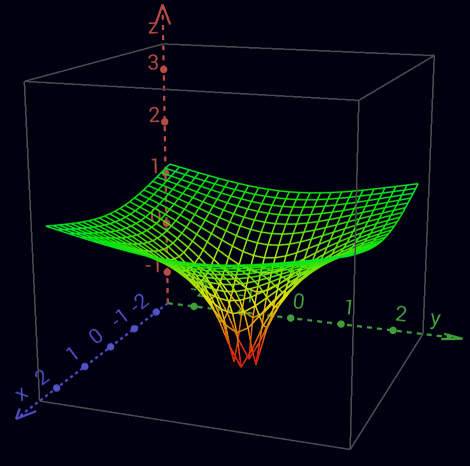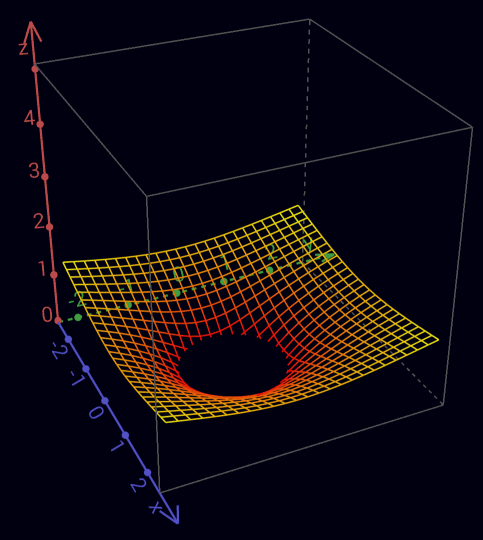Real part of the complex logarithm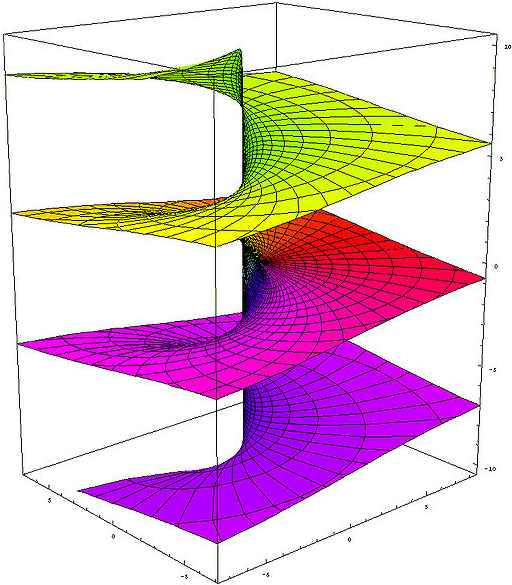Imaginary part of the complex logarithm - Riemann surface

Absorption
([a]∎) =      Zero cardinality of a             Theorem 3   a * 0 = 0

Proof of Zero cardinality of a
([a]∎)
([a]∎)([a][b])<([a][b])>   -Inversion of ([a][b])
([a][b])<([a][b])>   -Distribution of [a]
Inversion of ([a][b])

Indefiniteness of cardinality of ∎ within ()   0 = eln 0 = 0 * 0 = 0 * 0 * 0 = ...
= (∎) = (∎∎) = (∎∎∎) = (∎∎∎∎) = ...    -Involution2, -Zero cardinality of void

= <> = [o] = (∎) = [(<>)] = ([<>]) =  <[o]> = <(∎)> 5x Inversion of void, 3x Involution1, 3x Involution2

Indefiniteness of Inversion of ∎ within ()
(<∎>) = ([o]<∎>) <= 1/0 is undefined (singularity)

0 * ? = 1   zero don't have multiplicative inverse
0/0 ambiguous
0/a = 0   a/a = 1   a/0 = ∞

Cardinality of [a] (aggregation third degree)
([a]...[a]) = (([[a]][o...o]))
(([[a]][b]))      ab

Due to the complete ambiguity in the imaginary part of ln 0, ∎ is defined besides the zero cardinality only at a 0-basis.

0n = 0   n ∈ ℕ \ 0
(([∎][o...n]))
(     ∎...n  )   cardinality von ∎
(     ∎      )   Indefiniteness of cardinality von ∎
Involution2

0q = 0   q ∈ ℚ \ 0
((    [∎][      q       ]))
((    [∎][([m]    <[n]>)]))    m, n ∈ ℕ \ 0
((    [∎]  [m]    <[n]>  ))    Involution1
(([[(([∎]  [m]))]]<[n]>  ))    2x -Involution1
(([[            ]]<[n]>  ))    0m = 0
((    [∎]         <[n]>  ))    Indefiniteness of cardinality von ∎
((    [∎]       [(<[n]>)]))    -Involution1
Zero cardinality of (<[n]>)

00 = 1
(([∎]∎))
(      )   Zero cardinality of ∎

a0 = 1
(([[a]]∎))
(        )   Zero cardinality of [a]

a1 = a
(([[a]][o]))
(([[a]]   ))   Involution1
a          2x Involution2

Since multiplication is as commutative as addition, factors can be aggregated just like summands.

Addition         2 + 4                 = 6
Multiplikation   2 * 4 = 2 + 2 + 2 + 2 = 8
Exponentiation   24    = 2 * 2 * 2 * 2 = 16
Tetration        42   = 2222 = 224 = 216 = 65.536
Hyper-5          24 = 2222 = 422 = 65.5362 = 2...2 65.536 times - therefore, our universe is far too small
...

Since exponentiation is not commutative, exponents cannot be represented aggregated like summands or factors.
Thus aggregations lose their beauty and thus their usefulness from here on.
oo     ooo                                   2 + 3             = 5
(  [oo]   [ooo] )            =   oo  oo  oo      2 * 3 = 2 + 2 + 2 = 6
(([[oo]]  [ooo]))            = ([oo][oo][oo])    23    = 2 * 2 * 2 = 8
(([[oo]][ (([[oo]][oo])) ])) = (([[oo]][oooo]))  32    = 222  = 24  = 16
(([[oo]][ (([[oo]][ (([[oo]][oo])) ])) ]))       23 = 222 = 42 = 2222 = 65.536
...

2+2 = 2*2 = 22 = 22 = 22 = ... = 4

Inversion
( [<a>]b  ) = <( [a]b  )>    Inverse Promotion1      Theorem 4   -a * eb = -(a * eb)
(<[<a>]b>c) = <(<[a]b>c)>    Inverse Promotion2      Theorem 5   1/-a * eb = -(1/a * eb)

Proof of Inverse Promotion1
([<a>]b)
<([a]b)>([a]b)([<a>]b)    -Inversion of ([a]b)
<([a]b)>([a     <a>]b)    -Distribution von b
<([a]b)>([         ]b)    Inversion of a
<([a]b)>([         ] )    Zero cardinality of (b)
<([a]b)>                  Involution2

Proof of Inverse Promotion2
(c<b    [<a>]>                )
(c<b    [<a>]><[<d>]    >[<d>])     -Inversion of [<d>]
<(c<b    [<a>]><[<d>]    >[ d ])>    Inverse Promotion1
<(c<b    [<a>]  [<d>]    >[ d ])>    Inverse Collection
<(c<b[  ([<a>]  [<d>])  ]>[ d ])>    -Involution1
<(c<b[<<([ a ]  [ d ])>>]>[ d ])>    2x Inverse Promotion1
<(c<b[  ([ a ]  [ d ])  ]>[ d ])>    Inverse Cancellation
<(c<b    [ a ]  [ d ]    >[ d ])>    Involution1
<(c<b    [ a ]><[ d ]    >[ d ])>    -Inverse Collection
<(c<b    [ a ]>                )>    Inversion of [d]

Operators
-a => <a>
a+b => ab
a+b+c => abc
a-b => a<b>
a*b => ([a][b])
a*b*c => ([a][b][c])
a*-b => ([a][<b>]) = <([a][b])> = ([<a>][b])      +-Inverse Promotion1
a/b => ([a]<[b]>)                                                          for b !=
1/b => ([o]<[b]>) = (<[b]>)                      Involution1               for b !=
a/a => ([a]<[a]>) = o                            Inversion of [a]          for a !=
ab  => (([[a]][b]))
a1  => (([[a]][o])) = (([[a]])) = a              Involution1, 2x Involution2
a-b => (([[a]][<b>]))
a1/b => (([[a]]<[b]>))    = (([[a]][(<[b]>)]))    -Involution1               for b !=
ac/b => (([[a]]<[b]>[c])) = (([[a]][(<[b]>[c])])) -Involution1               for b !=

abc
(([[a]][(([[b]][c]))]))
(([[a]]  ([[b]][c])  ))   Involution1

((ab)c)1/d = ab * c / d
(([[(([[(([[a]][b]))]][c]))]]<[d]>))
((        [[a]][b]    [c]    <[d]>))   4x Involution1

(1/a)b = a-b
( ([[(<[a]>)]][ b ]) )
( ([  <[a]>  ][ b ]) )   Involution1
(<([   [a]   ][ b ])>)   Inverse Promotion1
( ([   [a]   ][<b>]) )   -Inverse Promotion1

ab * ac = ab + c
([(([[a]][b]))][(([[a]][c]))])
(  ([[a]][b])    ([[a]][c])  )   2x Involution1
(  ([[a]][b             c])  )   Distribution of [[a]]

ac * bc = (a * b)c
([(([[a]][c]))][(([[b]][c]))])
(  ([[a]][c])    ([[b]][c])  )   2x Involution1
(  ([[a]           [b]][c])  )   Distribution of [c]

1 / e = e-1
([o]<[(o)]>)
(   <[(o)]>)
(   <  o  >)    2x Involution1

1 / e2 = e-2
([o]<[(oo)]>)
(   <[(oo)]>)
(   <  oo  >)   2x Involution1

ln (a * b) = ln a + ln b
[([a][b])]
[a][b]      Involution1

ln (a / b) = ln a - ln b
[([a]<[b]>)]
[a]<[b]>      Involution1

ln (ab) = ln a * b
[(([[a]][b]))]
([[a]][b])      Involution1

logb a = ln a/ln b
([[a]]<[[b]]>)

1/(1/a) = a
(<[(<[a]>)]>)
(<  <[a]>  >)   Involution1
(    [a]    )   Inverse Cancellation
a         Involution2

(1/a)*(1/b) = 1/(a*b)
([(<  [a]>)][(<[b]  >)])
(  <  [a]>    <[b]  >  )   2x Involution1
(  <  [a]      [b]  >  )   Inverse Collection
(  <[([a]      [b])]>  )   -Involution1

a/c + b/d = (a*d + b*c) / c*d
(  [a]          <[c]>)(  [b]          <[d]>)
(  [a][d]  <[d]><[c]>)(  [b][c]  <[c]><[d]>)   -Inversion of [d] and [c]
(  [a][d]  <[d]  [c]>)(  [b][c]  <[c]  [d]>)   2x Inverse Collection
([([a][d])]<[d]  [c]>)([([b][c])]<[c]  [d]>)   2x -Involution1
([([a][d])              ([b][c])]<[c]  [d]>)   -Distribution of <[c][d]>

1/a + 1/b = (a + b) / a*b
(        <[a]>)(        <[b]>)
([b]<[b]><[a]>)([a]<[a]><[b]>)   -Inversion of [b] and [a]
([b]<[b]  [a]>)([a]<[a]  [b]>)   2x Inverse Collection
([ab]<[a][b]>)                   -Distribution of <[a][b]>

(a + b) * (a - b) = a² - b²
([a       b][a                    <b>])
([a       b][a]) ([a           b][<b>])    Distribution of [ab]
([a][a])([b][a]) ([a][<b>])  ([b][<b>])    Distribution of [a] and [<b>]
([a][a])([b][a])<([a][ b ])><([b][ b ])>   2x -Inverse Promotion1
([a][a])                    <([b][ b ])>   Inversion of ([a][b])
(([[a]][oo]))            <(([[b]][oo]))>   Cardinality of [a] and [b]

Numbers
0 =>
1 => o
2 => oo
-1 => <o>
-2 => <oo>
1/2 => (<[oo]>)
2/3 => ([oo]<[ooo]>)
43 => ([b][oooo])ooo             for b = oooooooooo
243 => ([b][([b][oo])oooo])ooo    for b = oooooooooo
1243 => ([b][([b][boo])oooo])ooo   for b = oooooooooo

Arity
{a} = ([oooooooooo][a])   Definition of {}
oooooooooo{a} = {o a}     Carry+            Cardinality of oooooooooo
{a}{b} = {ab}             Collection+
([{a}]b) = {([a]b)}       Promotion+
{} =                      Zero cardinality+

23 * 114 = 2622
([ {oo}ooo ] [ {{o}o}oooo ])
([{oo}][{{o}o}oooo])  ([ooo][{{o}o}oooo])              Distribution of [{{o}o}oooo]
{([ oo ][{{o}o}oooo])} ([ooo][{{o}o}oooo])              Promotion+
{{{o}o}oooo{{o}o}oooo} {{o}o}oooo{{o}o}oooo{{o}o}oooo   2x -Cardinality of {{o}o}oooo
{{{o}o}oooo{{o}o}oooo} {{o}o}{{o}o}{{o}o}oooooooooooo   Collection+ of o
{{{o}o}oooo{{o}o}oooo} {{o}o}{{o}o}{{o}oo}         oo   Carry+
{{{o}o}    {{o}o} {o}{o}{o}  oooooooooooo}         oo   Collection+ of {o}
{{{o}o}    {{o}o} {o}{o}{oo}           oo}         oo   Carry+
{{{o}       {o}      oooooo}           oo}         oo   Collection+ of {{o}}
{{{         oo}      oooooo}           oo}         oo   Collection+ of {{{o}}}

Inverse Arity
/a\ = (<[oooooooooo]>[a])   Definition of /\
/oooooooooo a\ = o/a\       Carry-            Cardinality of oooooooooo
/a\/b\ = /ab\               Collection-
([/a\]b) = /([a]b)\         Promotion-
/\ =                        Zero cardinality-
{/a\} = /{a}\ = a           Arity Cancellation

12,1 * 1,012 = 12,2452
([ {o}oo/o\ ] [ o//o/oo\\\ ])
([{o}][o//o/oo\\\]) ([oo][o//o/oo\\\]) ([/o\][o//o/oo\\\])    2x Distribution of [o//o/oo\\\]
{([ o ][o//o/oo\\\])}([oo][o//o/oo\\\])/([ o ][o//o/oo\\\])\   2x Promotion ±
{       o//o/oo\\\  } o//o/oo\\\o//o/oo\\\    /o//o/oo\\\  \   3x -Cardinality of o//o/oo\\\
{o}{     //o/oo\\\  } o//o/oo\\\o//o/oo\\\    /o//o/oo\\\  \   -Collection+
{o}       /o/oo\\     o//o/oo\\\o//o/oo\\\    /o//o/oo\\\  \   Arity Cancellation1
{o}oo     /o/oo\\      //o/oo\\\ //o/oo\\\    /o//o/oo\\\  \   Collection+ of o
{o}oo     /oo/oo\       /o/oo\\   /o/oo\\       //o/oo\\\  \   Collection- of /o\
{o}oo     /oo/oooo        /oo\      /oo\         /o/oo\\\  \   Collection- of //o\\
{o}oo     /oo/oooo        /ooooo                   /oo\\\  \   Collection- of ///o\\\

100 / 3 = 33,3...
([ {{o}} ]<[ooo]>)
{([  {o}  ]<[ooo]>)}                                                Promotion+
{([oooooooooo]<[ooo]>)}                                             -Carry+
{([ooo]<[ooo]>)([ooo]<[ooo]>)([ooo]<[ooo]>)        ([o]<[ooo]>)}    3x Distribution of <[ooo]>
{(            )(            )(            )        ([o]<[ooo]>)}    3x Inversion of [ooo]
{ooo}                                             {([o]<[ooo]>)}    -Collection+
{ooo}                                            ([{o}]<[ooo]>)     -Promotion+
{ooo}                                     ([oooooooooo]<[ooo]>)     -Carry+
{ooo}([ooo]<[ooo]>)([ooo]<[ooo]>)([ooo]<[ooo]>)    ([o]<[ooo]>)     3x Distribution of <[ooo]>
{ooo}(            )(            )(            )    ([o]<[ooo]>)     3x Inversion of [ooo]
{ooo}ooo                                ([/oooooooooo\]<[ooo]>)     -Carry-
{ooo}ooo                               /([ oooooooooo ]<[ooo]>) \   Promotion-
{ooo}ooo/([ooo]<[ooo]>)([ooo]<[ooo]>)([ooo]<[ooo]>)([o]<[ooo]>) \   3x Distribution of <[ooo]>
{ooo}ooo/(            )(            )(            )([o]<[ooo]>) \   3x Inversion of [ooo]
{ooo}ooo/ooo                            ([/oooooooooo\]<[ooo]>) \   -Carry-
{ooo}ooo/ooo                           /([ oooooooooo ]<[ooo]>)\\   Promotion-
...

100 / 3,3 = 30,3030...
([ {{o}} ]<[ooo/ooo\]>)
{([  {o}  ]<[ooo/ooo\]>)}                                                 Promotion+
{([oooooooooo]<[ooo/ooo\]>)}                                              -Carry+
{([ooooooooo/ooo\/ooo\/ooo\/o\]<[ooo/ooo\]>)}                             -Carry- and 3x -Collection-
{([ooo/ooo\]<[ooo/ooo\]>)(dito)(dito)        ([ /o\ ]<[ooo/ooo\]>)}       3x Distribution of <[ooo/ooo\]>
{(                      )(    )(    )        ([ /o\ ]<[ooo/ooo\]>)}       3x Inversion of [ooo/ooo\]
{ooo}                                       {([ /o\ ]<[ooo/ooo\]>)}       -Collection+
{ooo}                                        ([{/o\}]<[ooo/ooo\]>)        -Promotion+
{ooo}                                        ([  o  ]<[ooo/ooo\]>)        Arity Cancellation1
{ooo}                                 ([/oooooooooo\]<[ooo/ooo\]>)        -Carry-
{ooo}                                /([ oooooooooo ]<[ooo/ooo\]>)    \   Promotion-
{ooo}                 /([ooooooooo/ooo\/ooo\/ooo\/o\]<[ooo/ooo\]>)    \   -Carry- and 3x -Collection-
{ooo}/([ooo/ooo\]<[ooo/ooo\]>)(dito)(dito)     ([/o\]<[ooo/ooo\]>)    \   3x Distribution of <[ooo/ooo\]>
{ooo}/(                      )(    )(    )     ([/o\]<[ooo/ooo\]>)    \   3x Inversion of [ooo/ooo\]
{ooo}/ooo                           ([//oooooooooo\\]<[ooo/ooo\]>)    \   -Carry-
{ooo}/ooo                         //([  oooooooooo  ]<[ooo/ooo\]>)  \\\   2x Promotion-
{ooo}/ooo            //([ooooooooo/ooo\/ooo\/ooo\/o\]<[ooo/ooo\]>)  \\\   -Carry- and 3x -Collection-
{ooo}/ooo//([ooo/ooo\]<[ooo/ooo\]>)(dito)(dito)([/o\]<[ooo/ooo\]>)  \\\   3x Distribution of <[ooo/ooo\]>
{ooo}/ooo//(                      )(    )(    )([/o\]<[ooo/ooo\]>)  \\\   3x Inversion of [ooo/ooo\]
{ooo}/ooo//ooo                      ([//oooooooooo\\]<[ooo/ooo\]>)  \\\   -Carry-
{ooo}/ooo//ooo                    //([  oooooooooo  ]<[ooo/ooo\]>)\\\\\   2x Promotion-
...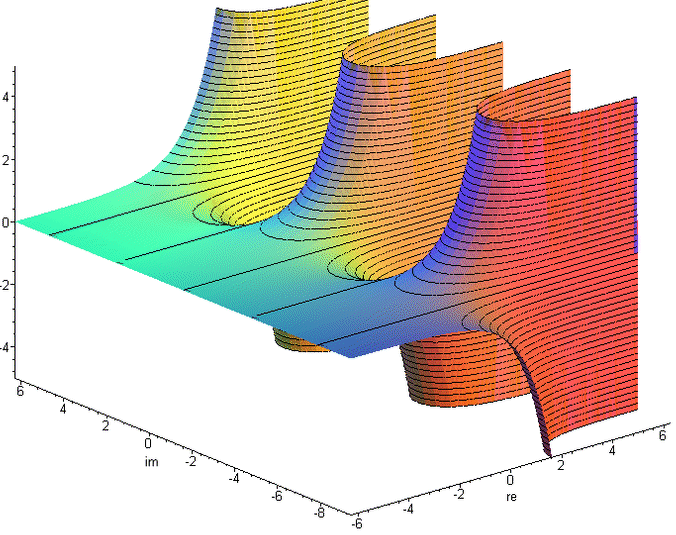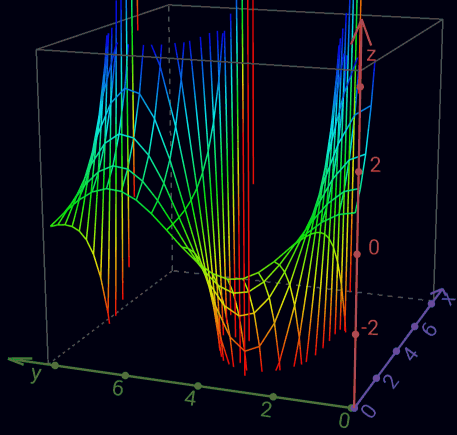Real part of the complex exponential function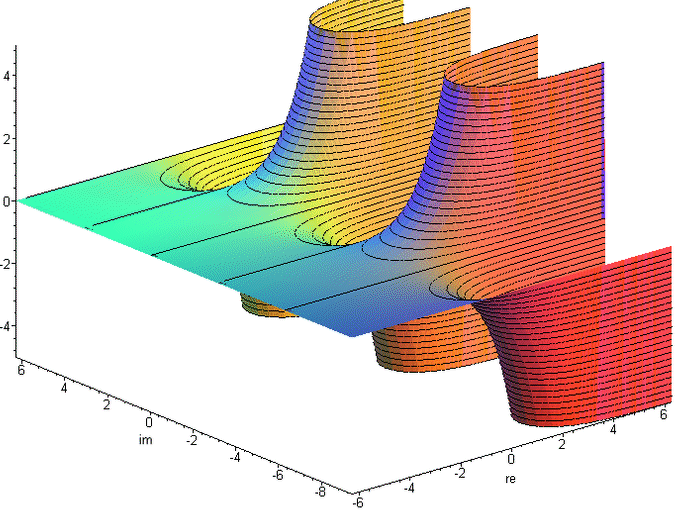Imaginary part of the complex exponential function

Transcendental
J :=  [<o>]                Phase Element            Definition   J := ln -1 = πi         (~3.1415926535i)
(J) = ([<o>]) = <o>         Involution2                           eJ = eπi = -1
(o)                         Euler number                          e1 = e                  (~2.71828182845)
((o))                                                             ee                      (~15.1542622414)
[<(a)>] = a[<o>]            Phase Independence of a  Theorem 6    ln -ea = a + ln -1
JJ =                       J-cancellation           Theorem 7
J = <J>                   Self-Inversion of J      Theorem 8
i := (([J]<[oo]>))         imaginary unit           Definition   i :=  √-1 = eJ/2
i = (<[<i>]>) = <(<[i]>)>   imaginary unit           Theorem 9    i = 1/-i = -e-ln i
([<J>][i]) = <([J][i])> = ([J][<i>]) = ([J]<[i]>)   Circle number Pi     π = -J*i = J*-i = J/i   (~3.1415926535)
(([[<o>]][<i>])) = (([[<o>]]<[i]>))             eπ = (-1)-i = (-1)1/i    (~23.1406926328)

Like ln in the imaginary, by an arbitrary multiple of 2π is ambiguous, so at e all values are repeated every 2πi.
To represent this, the J-cancellation and self-inversion of J is permissible only within an instantiation,
or they must be contained in at least one more instantiation than in abstractions.
Otherwise, a contradiction-producing proliferation of phase elements would be possible.

Proof of Phase Independence of a     for a != [∎]
[<(a)>              ]
[<(a)>( [         ])]  -Involution2
[<(a)>(a[         ])]  -Zero cardinality of (a)
[<(a)>(a[o     <o>])]  -Inversion of o
[<(a)>(a[o])(a[<o>])]  Distribution of a
[<(a)>(a   )(a[<o>])]  Involution1
[           (a[<o>])]  Inversion of (a)
a[<o>]    Involution1

Proof of J-cancellation
[<o>][<o>]
[<([<o>])>]   -Phase Independence von [<o>]
[<  <o>  >]   Involution2
[    o    ]   Inverse Cancellation
Involution1

Proof of Self-Inversion of J
J
J<    >   -Inversion of void
J<J  J>   -J-cancellation2
J<J><J>   -Inverse Collection
<J>   Inversion of J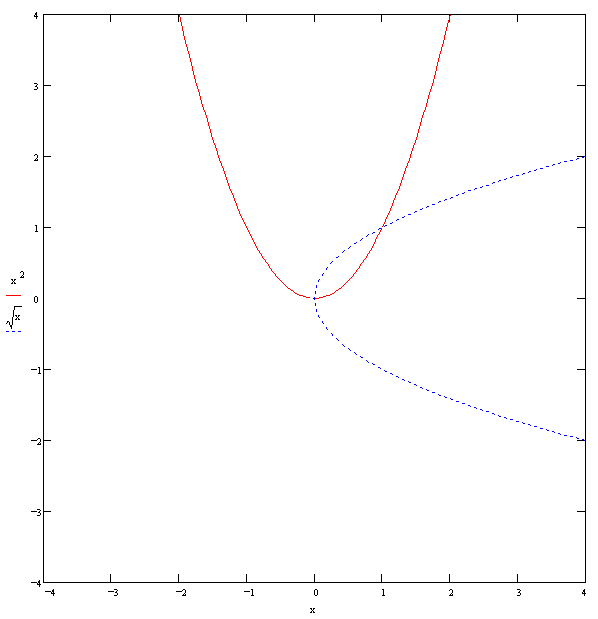f1(x) = x2
f2(x) = √x
f1(f2(x)) = x   (√x)2 = x
f2(f1(x)) = ±x   √(x2) = ±xReal part and imaginary part of the complex square root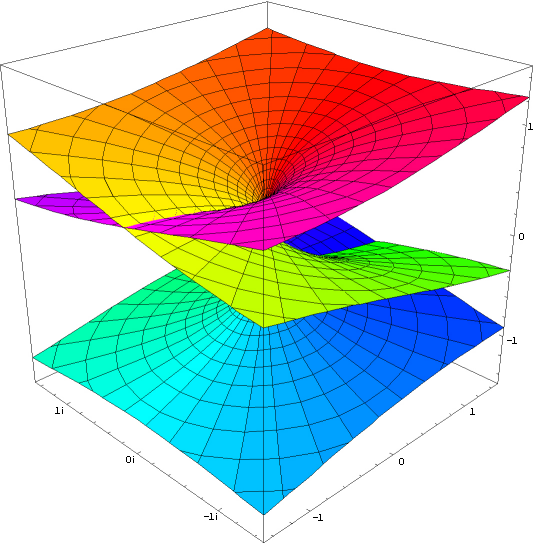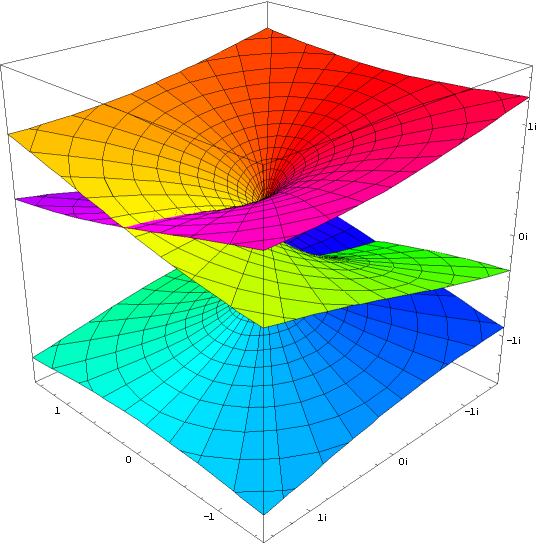Real part and imaginary part of the complex cube root

Whenever the exponent is a non-integer rational number, there is an ambiguity.
The ambiguity is equal to the (divisor alien) denominator.
If the exponent is less than 0, the base equals 0 gives a degenerate point.

i * i = i2 = -1
( [(([J]<[oo]>))] [(([J]<[oo]>))] )
(([ [(([J]<[oo]>))] ][oo]))       Cardinality of [(([J]<[oo]>))]
((     [J]<[oo]>     [oo]))       2x Involution1
((     [J]               ))       Inversion of [oo]
<o>                        2x Involution2

Proof of imaginary unit1
<(<  [i]>          )>
<(<  [i]><[i]  >[i])>    Inversion of [i]
<(<  [i]  [i]  >[i])>    Inverse Collection
<(<[([i]  [i])]>[i])>    -Involution1
<(<[    <o>   ]>[i])>    i * i = -1
<<(<[     o    ]>[i])>>   Inverse Promotion1
(              [i])     Inverse Cancellation, Involution1, Inversion of void
i       Involution2

-1 * -1 = 1
([<o>][<o>])
(          )   J-cancellation1

ee = ee
(([[(o)]][(o)]))
((         o  ))   3x Involution1

<  a  >  = ([<([a])>]) = ([a][<o>]) = ([a]J)   2x-Involution2, Phase Independence of [a]
[<  a  >] =  [<([a])>]  =  [a][<o>]  =  [a]J    -Involution2, Phase Independence of [a]
<( a )>  = ([<( a )>]) =   (a[<o>]) =   (aJ)   -Involution2, Phase Independence von  a

Indefiniteness of Cardinality of J     within ()
... = (<JJJJ>) = (<JJ>) =  o  = (JJ)  = (JJJJ)  = ...   J-cancellation, Inversion of void
... = (<JJJ>)  = (<J>)  = (J) = (JJJ) = (JJJJJ) = ...   J-cancellation, Self-Inversion of J

π * i = J
([ ([<J>][i]) ][i])
(   [<J>][i]   [i])     Involution1
(   [<J>][<o>    ])     i * i = -1
<<(   [ J ][ o     ])>>   2x Inverse Promotion1
J                 Inverse Cancellation, Involution1, Involution2

J/i = π * i / i = π
([      J            ]<[i]>)
([([ ([<J>][i]) ][i])]<[i]>)    J = π * i
(     [<J>][i]   [i]  <[i]>)    2x Involution1
(     [<J>][i]             )    Inversion of [i]

ii = eJ*i/2 = e-π/2
(([[ (([J]<[oo]>)) ]][i]))
((     [J]<[oo]>     [i]))   2x Involution1

i-2i = eπ
(([[ (([J]<[oo]>)) ]][<i>][oo]))
((     [J]<[oo]>     [<i>][oo]))   2x Involution1
((     [J]           [<i>]    ))   Inversion of [oo]

1/√a = √(1/a)
(<[(([ [a] ]<[oo]>))]>)
(<  ([ [a] ]<[oo]>)  >)   Involution1
(   ([<[a]>]<[oo]>)   )   -Inverse Promotion1

√9 = √±32 = ±3
(([[   ooooooooo   ]]<[oo]>))
(([[(  [ooo][ooo] )]]<[oo]>))   Cardinality of ooo
(([[(([[ooo]][oo]))]]<[oo]>))   Cardinality of [ooo]
((    [[ooo]][oo]    <[oo]>))   2x Involution1
((    [[ooo]]              ))   Inversion of [oo]
ooo                     2x Involution2
and
(([[       ooooooooo      ]]<[oo]>))
(([[  (  [ ooo ][ ooo ])  ]]<[oo]>))   Cardinality of ooo
(([[<<(  [ ooo ][ ooo ])>>]]<[oo]>))   -Inverse Cancellation
(([[  (  [<ooo>][<ooo>])  ]]<[oo]>))   2x -Inverse Promotion1
(([[  (([[<ooo>]][oo]) )  ]]<[oo]>))   Cardinality of [<ooo>]
((      [[<ooo>]][oo]       <[oo]>))   2x Involution1
((      [[<ooo>]]                 ))   Inversion of [oo]
<ooo>                        2x Involution2

(√3)2 = 3
(([[ (([[ooo]]<[oo]>)) ]][oo]))
((     [[ooo]]<[oo]>     [oo]))   2x Involution1
((     [[ooo]]               ))   Inversion of [oo]
ooo                      2x Involution2

-22 = 22
(([[<oo>]][oo]))
([<oo>][<oo>])     -Cardinality of [<oo>]
<<([ oo ][ oo ])>>   2x Inverse Promotion1
([ oo ][ oo ])     Inverse Cancellation
(([[oo]][oo]))       Cardinality of [oo]

ab = ((±a2)1/2)b = -ab
((      [[ a ]]                 [b]))
((      [[ a ]][oo]       <[oo]>[b]))   -Inversion of [oo]             !!! Double entendre
(([[  (([[ a ]][oo]))  ] ]<[oo]>[b]))   2x -Involution1
(([[  (  [ a ][ a ] )  ] ]<[oo]>[b]))   -Cardinality of [a]
(([[<<(  [ a ][ a ] )>>] ]<[oo]>[b]))   -Inverse Cancellation
(([[  (  [<a>][<a>] )  ] ]<[oo]>[b]))   2x -Inverse Promotion
(([[  (([[<a>]][oo]))  ] ]<[oo]>[b]))   Cardinality of [<a>]
((      [[<a>]][oo]       <[oo]>[b]))   2x Involution1
((      [[<a>]]                 [b]))   Inversion of [oo]Three-dimensional representation of the Eulerian formula ez*i = cos z + i sin z   =>  (([z]([J]<[oo]>)))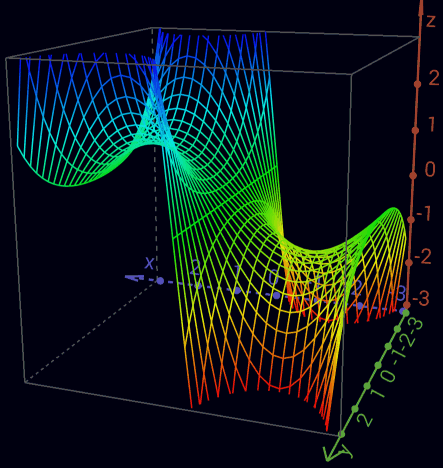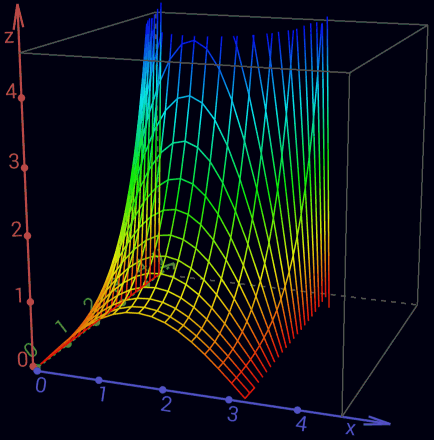Real part of the complex sine function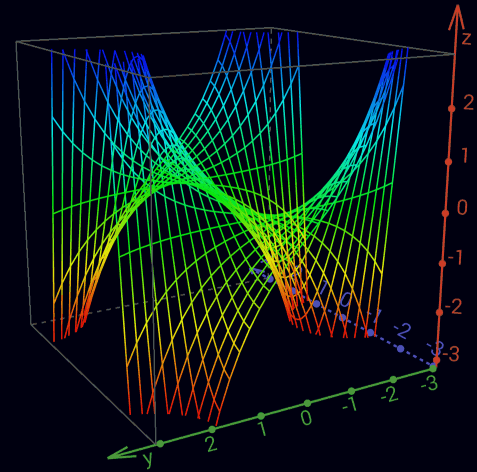Imaginary part of the complex sine function

an+1 =  ([an]J)      o -> (J) -> o -> (J) -> o -> ...  <=  (1 -> -1 ->  1 -> -1 -> 1 -> ...)
an+1 =  ([an][i])    o -> i -> (J) -> <i> -> o -> ...  <=  (1 ->  i -> -1 -> -i -> 1 -> ...)
an+1 = <([an][i])>   o -> <i> -> (J) -> i -> o -> ...  <=  (1 -> -i -> -1 ->  i -> 1 -> ...)

ea*i = cos a + i sin a    =>     (([a][i]))
e-a*i = cos a - i sin a   =>                 (([<a>][i]))
sin a = (ea*i - e-a*i)/2i  =>  ([ (([a][i]))<(([<a>][i]))>]<[oo][i]>)
cos a = (ea*i + e-a*i)/2   =>  ([ (([a][i])) (([<a>][i])) ]<[oo]   >)

ea  = cosh a + sinh a   =>     (a)
e-a = cosh a - sinh a   =>         (<a>)
sinh a = (ea - e-a)/2   =>   ([(a)<(<a>)>]<[oo]>)
cosh a = (ea + e-a)/2   =>   ([(a) (<a>) ]<[oo]>)

sin(x + i y) = sin(x) cosh(y) + i cos(x) sinh(y)
cos(x + i y) = cos(x) cosh(y) - i sin(x) sinh(y)
sin(z) = -i sinh(i z)
sinh(z) =  -i sin(i z)
cos(z) =    cosh(i z)
cosh(z) =     cos(i z)
sin'(z) =  cos(z)
sinh'(z) = cosh(z)
cos'(z) = -sin(z)
cosh'(z) = sinh(z)

unit circle    x2 + y2 = 1   (([[x]][oo])) (([[y]][oo]))  = o
unit hyperbole x2 - y2 = 1   (([[x]][oo]))<(([[y]][oo]))> = o
```